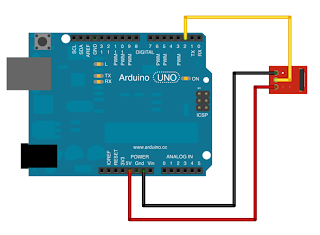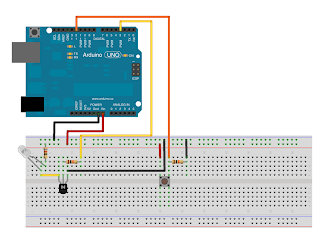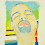## Saturday, October 6, 2012

Attending Geekcon last month really energized me to get to do more "physical" kind of things. We built an arduino based drawing robot. An instructable was posted about it here: http://www.instructables.com/id/Artibot-a-portrait-painting-robot/

[Edit: part two of the story is available here: http://srooltheknife.blogspot.co.il/2012/10/arduino-adventures-part-ii.html]

So, I was thinking of a next project, and this idea came up - the weather here is quite hot and humid this time of year, so often I go to sleep with the air condition on. This is very wasteful and in addition, makes my wife environment too cold for her taste (is it true that women are often feeling more cold than men?).
So the idea was to make an arduino talk to the air condition mimicking its remote control, allowing me to turn the air condition for 10minutes, get the room pleasant, and while sleeping make the arduino kick the aircon on for 10 minutes or so every hour to keep the room pleasant (but not freezing cold).

So, got the following items to get going:
1. Arduino Uno board
2. IR sensor - TSOP382 from Vishay (if you are Israeli, this is an excellent source: http://www.4project.co.il/product/757?sectid=79). This is a very cool sensor that filters the IR modulation frequency used by the remote (38kHz) and gives you a clean signal to work with
3. IR led - http://www.4project.co.il/product/868

I started with trying to decode my existing air-con remote control signals. Here is the hardware setup I used for this (very simple... the item on the right is the TSOP382 withits input going to pin 2):Circuit 1 - IR remote sensor circuit

This was much harder than I expected...
I started with this code:
http://www.instructables.com/id/Clone-a-Remote-with-Arduino/
simply recording the stream of signals
I assume playing this sequence back through the IR diode (modulating to 38 kHz) will work. But I first wanted to really understand the logic behind this sequence.
This proved to be quite difficult to begin with. First step forward was when I found this great library for reading all kind of IR remote protocols made by Ken Shirrif: http://www.arcfn.com/2009/08/multi-protocol-infrared-remote-library.html
I was disappointed to find that it does not recognise my air-con remote at all. But it did recognise my Apple TV remote, so it gave me some hope...
I decided I need some way to visualize the remote signal. So I wrote this little sketch:

``````#include <IRremote.h>

int RECV_PIN = 2;
int BUTTON_PIN = 12;
int STATUS_PIN = 13;

IRrecv irrecv(RECV_PIN);
IRsend irsend;

decode_results results;

void setup()
{
Serial.begin(9600);
pinMode(BUTTON_PIN, INPUT);
pinMode(STATUS_PIN, OUTPUT);
}

// Storage for the recorded code
int codeType = -1; // The type of code
unsigned long codeValue; // The code value if not raw
unsigned int rawCodes[RAWBUF]; // The durations if raw
int codeLen; // The length of the code
int toggle = 0; // The RC5/6 toggle state

void printCodeWave() {
char ch;
for (int i = 0 ; i < codeLen ; i++) {
if (i % 2) {
ch = '_';
}
else {
ch = '^';
}
int len = ceil(rawCodes[i] / 300.0);
for (int j = 0 ; j < len ; j++) {
Serial.print(ch);
}
}
Serial.println(";");
}

void printCodeNumeric() {
char ch;
for (int i = 0 ; i < codeLen ; i++) {
if (i % 2) {
Serial.print("s");
}
else {
Serial.print("m");
}
Serial.print(rawCodes[i], DEC);
Serial.print(", ");
}
Serial.println(";");
}

void printCode() {
boolean have_half = false;
boolean half_val = false;
for (int i = 0 ; i < codeLen ; i++) {
boolean isHigh = ((i % 2) == 0);
if (rawCodes[i] > 3400) {         //--------------- sync mark + half bit
Serial.print("<SYN+>");
have_half = true;
half_val = isHigh;
}
else if (rawCodes[i] > 2400) {    //--------------- sync mark with no extra
Serial.print("<SYN>");
have_half = false;
}
else if (rawCodes[i] > 1400) {    //--------------- double len
if (half_val) {
Serial.print("0");
}
else {
Serial.print("1");
}
have_half = true;
half_val = isHigh;
}
else {
if (have_half) {                //--------------- last in pair
have_half = false;
if (half_val) {
Serial.print("0");
}
else {
Serial.print("1");
}
}
else { // first in pair
have_half = true;
half_val = isHigh;
}
}

} // end loop

Serial.println(";");
printCodeNumeric();
printCodeWave();

}

// Stores the code for later playback
// Most of this code is just logging
void storeCode(decode_results *results) {
codeType = results->decode_type;
int count = results->rawlen;

codeLen = results->rawlen - 1;
// To store raw codes:
// Drop first value (gap)
// Convert from ticks to microseconds
// Tweak marks shorter, and spaces longer to cancel out IR receiver distortion
for (int i = 1; i <= codeLen; i++) {
if (i % 2) {
// Mark
rawCodes[i - 1] = results->rawbuf[i]*USECPERTICK - MARK_EXCESS;
}
else {
// Space
rawCodes[i - 1] = results->rawbuf[i]*USECPERTICK + MARK_EXCESS;
}
}
codeValue = results->value;

}

void loop() {
if (irrecv.decode(&results)) {
digitalWrite(STATUS_PIN, HIGH);
storeCode(&results);
printCode();
digitalWrite(STATUS_PIN, LOW);
}
}

``````

Opening the serial monitor and clicking the temperature down button twice, gave me this:

``````
<SYN><SYN>0001000000010110000000000000000010<SYN><SYN>0001000000010110000000000000000010<SYN><SYN>;
m3000, s3050, m900, s1000, m850, s1100, m850, s2050, m1800, s1100, m850, s1050, m900, s1050, m850, s1050, m850, s1150, m800, s1100, m850, s2000, m1850, s2000, m900, s1100, m1750, s1150, m850, s1000, m900, s1100, m800, s1100, m850, s1050, m850, s1100, m900, s1000, m900, s1100, m800, s1100, m850, s1100, m850, s1050, m850, s1050, m900, s1050, m850, s1050, m850, s1150, m800, s1100, m850, s2050, m1800, s1100, m2800, s3100, m850, s1050, m850, s1100, m850, s2000, m1900, s1000, m900, s1050, m850, s1100, m850, s1100, m800, s1100, m850, s1050, m850, s2100, m1800, s2000, m850, s1100, m1850, s1050, m850, s1100, m800, s1100, m850, s1050, m900, s1100, m850, s1050, m900, s1000, m850, s1100, m850, s1050, m900, s1050, m850, s1100, m850, s1050, m850, s1100, m850, s1100, m800, s1100, m900, s1000, m900, s2000, m1850, s1050, m2900, s3000, m900, ;
^^^^^^^^^^___________^^^____^^^____^^^_______^^^^^^____^^^____^^^____^^^____^^^____^^^____^^^_______^^^^^^^_______^^^____^^^^^^____^^^____^^^____^^^____^^^____^^^____^^^____^^^____^^^____^^^____^^^____^^^____^^^____^^^____^^^____^^^____^^^_______^^^^^^____^^^^^^^^^^___________^^^____^^^____^^^_______^^^^^^^____^^^____^^^____^^^____^^^____^^^____^^^_______^^^^^^_______^^^____^^^^^^^____^^^____^^^____^^^____^^^____^^^____^^^____^^^____^^^____^^^____^^^____^^^____^^^____^^^____^^^____^^^____^^^_______^^^^^^^____^^^^^^^^^^__________^^^;

<SYN><SYN>0001000000010100000000000000000010<SYN><SYN>0001000000010100000000000000000010<SYN><SYN>;
m3000, s3050, m850, s1050, m850, s1100, m800, s2100, m1800, s1100, m850, s1050, m900, s1000, m850, s1100, m850, s1100, m800, s1150, m850, s2000, m1800, s2100, m1850, s1000, m850, s1100, m850, s1050, m850, s1100, m850, s1100, m800, s1100, m900, s1050, m800, s1100, m850, s1100, m850, s1100, m800, s1100, m850, s1100, m850, s1050, m900, s1000, m850, s1100, m850, s1100, m850, s1050, m850, s2050, m1800, s1150, m2850, s3050, m800, s1100, m850, s1100, m850, s2050, m1800, s1100, m800, s1100, m850, s1100, m800, s1100, m850, s1100, m800, s1100, m950, s1950, m1800, s2050, m1900, s1000, m850, s1050, m850, s1100, m850, s1100, m800, s1100, m850, s1100, m850, s1050, m850, s1100, m850, s1100, m850, s1100, m800, s1100, m800, s1100, m900, s1050, m850, s1050, m850, s1100, m850, s1050, m950, s1000, m850, s2050, m1850, s1050, m2850, s3050, m900, ;
^^^^^^^^^^___________^^^____^^^____^^^_______^^^^^^____^^^____^^^____^^^____^^^____^^^____^^^_______^^^^^^_______^^^^^^^____^^^____^^^____^^^____^^^____^^^____^^^____^^^____^^^____^^^____^^^____^^^____^^^____^^^____^^^____^^^____^^^____^^^_______^^^^^^____^^^^^^^^^^___________^^^____^^^____^^^_______^^^^^^____^^^____^^^____^^^____^^^____^^^____^^^^_______^^^^^^_______^^^^^^^____^^^____^^^____^^^____^^^____^^^____^^^____^^^____^^^____^^^____^^^____^^^____^^^____^^^____^^^____^^^____^^^^____^^^_______^^^^^^^____^^^^^^^^^^___________^^^;

``````

In the output avobe, the first raw (starting with <syn>) shows my attempt at decoding the bits, the second raw is the numeric times of marks and spaces, and the bottom one is a graphical view which helped me a lot (trying to understand from the times the code was impossible).

Seing that bit string and assuming the whole remote control status is transmitted, I was able to decode what each bit (or the important ones) mean.

This is what I was able to make of it:

``````normal mode:
| p | mmm | ff | 0HV00 | tttt |0000 0000 0000 0000 010 |

I feel mode:
| p | mmm | ff | 1HV11 | tttt |0000 0000 0000 0000 010 |

``````

each character above is a bit where:
p = 1 when the power button is pressed (same message for on and off) ; 0  for any other button
mmm = mode where 001 = cool, 010 = heat, 011 is a drop (not sure what this means), 100 is fan only, 101 is "recycle" symbol (not sure what this means either).
ff = fan speed where 0 is low, 1 is medium, 2 is high and 3 is auto
H and V are the vertical and horizontal sweeping blades state
tttt = Temperature in degrees celsius - 15 (i.e. 0010 = 17, 0110 = 21, etc.)

At this stage I was ready to try and control my air condition. I used a 2n3904 transistor to switch on the IR LED (after finding out that it is a bad idea to drive it directly from the Arduino pin). Here is the hardware setup:Circuit 2 - IR LED push button transmitter

It has a push button connected to pin 12 (with a 10k resistor to ground), and pin 3 driving with PWM the IR LED via a 10k resistor going to the base of the 2N3904 transistor and an 82 ohm resistor to limit the current to the LED.

Started experimenting with the following sketch which takes a command according to the bit fields I explained above (in this case an on/off command with temperature set at 24 degrees and fan speed at low).

``````#include <IRremote.h>

int BUTTON_PIN = 12;
int STATUS_PIN = 13;

IRsend irsend;

decode_results results;

void setup()
{
Serial.begin(9600);
pinMode(BUTTON_PIN, INPUT);
pinMode(STATUS_PIN, OUTPUT);
}

unsigned int rawCodes[RAWBUF]; // The durations if raw
int codeLen; // The length of the code

unsigned char turnOnOffCmd = {
1,         // power on
0, 0, 1,   // cool (010 - heat, 011 - drop, 100 - fan, 101 - circ)
0, 0,      // fan (00 - low, 01 - med, 10 - high, 11 - auto)
0,         // i feel off (1 - on)
0,         // horiz vents movement
0,         // up-down vents movement
0, 0,
1, 0, 0, 1,  // 24 deg = T(C) - 15
0, 0, 0, 0,
0, 0, 0, 0,
0, 0, 0, 0,
0, 0, 0, 0,
0, 1, 0
};

int pulseWidthM = 1000;
int pulseWidthS = 950;

void sendCode(int repeat) {
codeLen = 0;
rawCodes[codeLen++] = 2900;  // sync high
rawCodes[codeLen++] = 2900;  // sync low

unsigned char lastVal = 0;
for (int i = 0 ; i < 34 ; i++) {
unsigned char bit = turnOnOffCmd[i];
if (bit) {
trail = 1;
trailW = pulseWidthM;
}
else {
trail = 0;
trailW = pulseWidthS;
}
if (lead == lastVal) {  // add to last valuse and emit short for next
rawCodes[codeLen++] = trailW;
}
else {                  // emit two short pulse
rawCodes[codeLen++] = trailW;
}
lastVal = trail;
}
// Assume 38 KHz
irsend.sendRaw3Times(rawCodes, codeLen);

}

int lastButtonState;

void loop() {
// If button pressed, send the code.
if (lastButtonState == HIGH && buttonState == LOW) {
Serial.println("Released");
}

if (buttonState) {
Serial.println("Pressed, sending");
digitalWrite(STATUS_PIN, HIGH);
sendCode(lastButtonState == buttonState);
digitalWrite(STATUS_PIN, LOW);
delay(50); // Wait a bit between retransmissions
}
lastButtonState = buttonState;
}

``````

Note that in the code above I am using the IRRemote library of Ken Shirriff, but I am using a method not included with the library - sendRaw3Times. This is part of my attempts to make this thing work. So far it does not... I am stuck here, and I hope that the decoding part was useful enough for you. If any of the readers can help with that - it would be great if you add this to the comments section below. Otherwise, stay tuned to my continuing adventrures... I do not intend to stop here...

OK, made some progress (not there yet, but had lots of fun). see the second part of this here: http://srooltheknife.blogspot.co.il/2012/10/arduino-adventures-part-ii.html

And a message from our advertisers:

Toptal provides remote engineers and designers of high quality. I recommend them. Follow this link (full disclosure: this is my affiliate link):
https://www.toptal.com/#engage-honest-computer-engineers-today

1.Hi,

Thank you for a great post. I looked at the IR codes but could not figure out the logic till I saw this post.

I'm currently controlling my AC using Arduino but I use recorded row codes:
http://www.hthome.co.il/vt161827.%D7%A9%D7%9C%D7%99%D7%98%D7%94-%D7%91%D7%9E%D7%96%D7%92%D7%9F-%D7%90%D7%9C%D7%A7%D7%98%D7%A8%D7%94-%D7%93%D7%A8%D7%9A-%D7%A2%D7%99%D7%A0%D7%99%D7%AA-%D7%AA%D7%A6%D7%95%D7%92%D7%94

Avi,

aviwolf@yahoo.com

1.2.Hi,

Did you managed eventually to control your AC?
I applied your code and it works fine. I added a new method to the IR library as you did but for some reason it didn't work. Since I already modified the IR library to work with a much larger buffer (200 instead of the default which I think was 75) as the AC IR codes are very long, I used this buffer and filled with 3 copies of the code + added 3900 as the last value and this worked fine.
I had to store all my codes in PROGMEM as I needed a few and fill a buffer from the PROGMEM before sending the code. Now I can completely stop using this method and use much smaller codes.
Thanks

2.Hi,

Avi Wolf may you post the complete code and the final to capture the code of the remote control of air conditioning??!

Thanks

3.Hi,

Did you figure out what all those extra bits are at the end of the code? Could it be CRC bits or time (start time, stop time or current time)?

Thanks,

Ori

1.unfortunately no :-(

4.This comment has been removed by the author.

5.Hi,

I think my a/c is the same. At least the cod so far matches what you have written.
In the modes, the "drop" symbol is for a dehumidification mode. The "recycle" symbol is a mode where it will supposedly change between heating and cooling to maintain temperature (sounds wasteful).
After the four temperature bits there is one bit which I have not figured out yet. Then, the second bit is on/off for a "sleep" mode (it's a mode which is supposed to start the cooling and slowly raise the temperature over time, one degree every hour for three hours).
The last 2 bits are always 1, 0 for me.
The 16 bits after the sleep bit and before the final "1, 0" are for the timer functions. I think they represent the start and stop times relative to the current time (as displayed on the remote). The remote can set an on timer, an off timer or both. The resolution for the timers is 10 minutes.I haven't yet figured out how these 16 bits work but here are the examples so far:

On in 2 minutes, off in 12: 0000 0001 0000 0010
Off in 2 minutes, on in 12: 0000 0010 0000 0001
On and off in almost 24hrs (I set both for 1540 when the time was 1543): 1100 0000 1100 0000

Any ideas would be nice.

Ori

6.Great info Ori. I am a bit busy at work right now, but hope to get back to you soon after looking into this...

7.First 8 bits are "on" time.
Next 8 bits are "off" time.

In each set the first 5 bits are hours and the last 3 are tens of minutes.

The on and off times are relative to the current time on the remote.
If you set the time on the remote to say 1805 and the start time to 1810 then the a/c will actually start 5 minutes late.